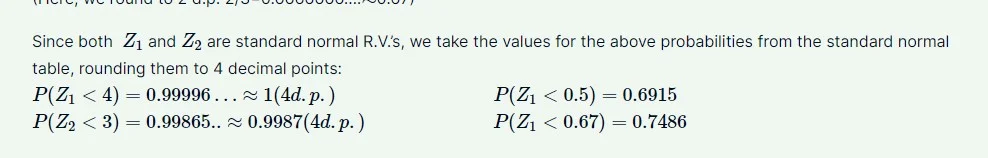22 Mar 2023

Posted on:

04 Jan 2023

0

# Practice Exam Question 2

I didn't understand why we get the probabilities from the standard normal distribution table as I get the same result but with using the PDF formula associated with a normal distribution. Would you please explain moreInstructor
Posted on:

05 Jan 2023

0

Hi Fatima,
The probabilities in the table are derived using the probability density function therefore the two correspond.
Best,
Ned

Posted on:

05 Jan 2023

0

What I get from what you say is that the values in the z-table are exactly the ones after computing the CDF for each value of z.
Please where can I find the z-table in the course ?

Thank you,

Posted on:

22 Mar 2023

0

The Z table is a std table available on the internet. Incidentally, the Z-table is also available in the Statistics course